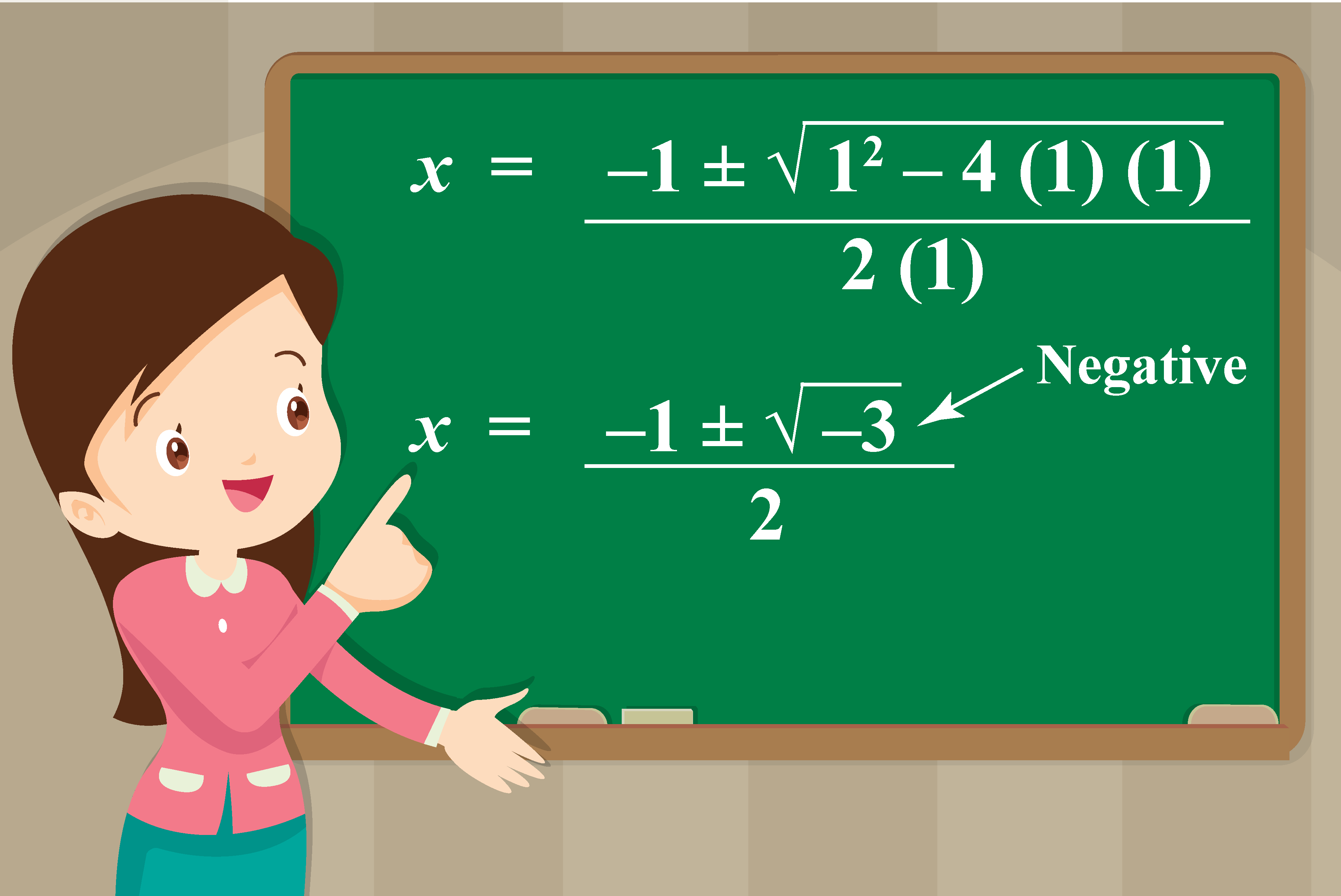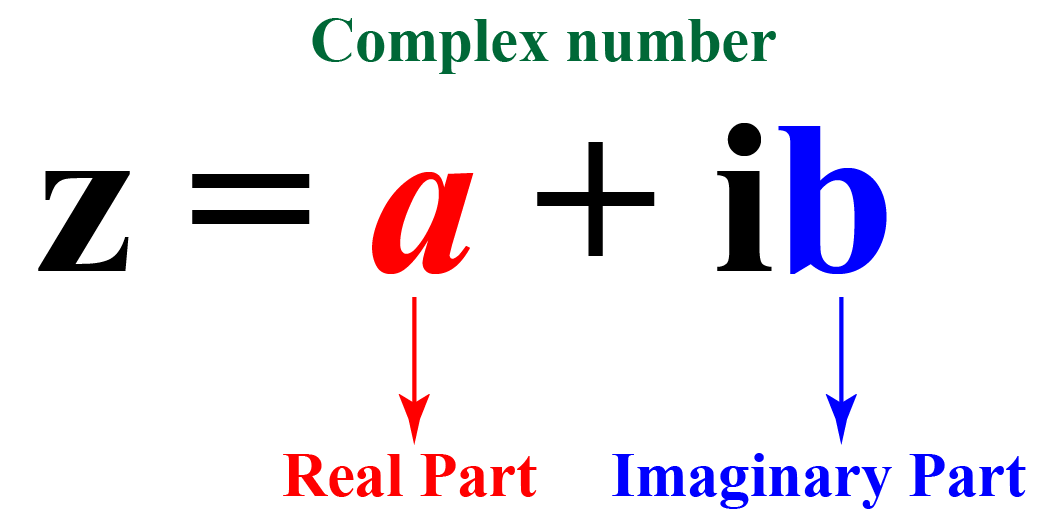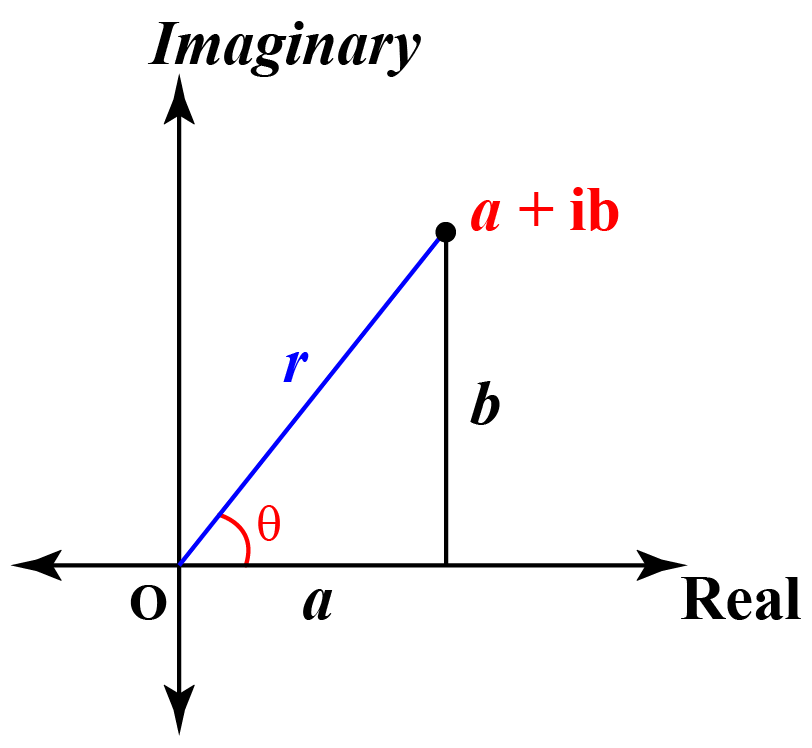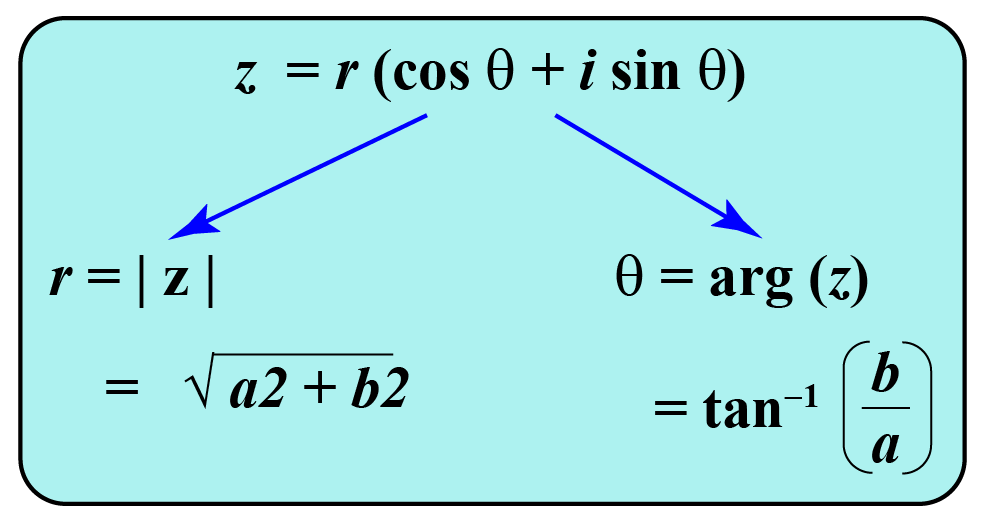# Division of complex Numbers

Division of complex Numbers

While doing this, sometimes, the value inside the square root may be negative.

For example, while solving a quadratic equation x2 + x + 1 = 0 using the quadratic formula, we get:So far we know that the square roots of negative numbers are NOT real numbers.

Then what type of numbers are they?

They are complex numbers

In this mini-lesson, we will learn about the division of complex numbers, division of complex numbers in polar form, the division of imaginary numbers, and dividing complex fractions. Check-out the interactive simulations to know more about the lesson and try your hand at solving a few interesting practice questions at the end of the page.

## Lesson Plan

 1 What Are Complex Numbers? 2 Important Notes 3 Solved Examples 4 Thinking Out of the Box! 5 Interactive Questions

## What Are Complex Numbers?

We denote $$\sqrt{-1}$$ by the symbol $$i$$ which we call "iota".

So, we have i2 = -1

This means you can say that $$i$$ is the solution of the quadratic equation x2 + 1 = 0

The number of the form z=a+ib, where $$a$$ and $$b$$ are real numbers are called the complex numbers.Here, $$a$$ is called the real part, and $$b$$ is called the imaginary part of the complex number $$z$$.

Division of complex numbers means doing the mathematical operation of division on complex numbers.

### Complex Numbers in Polar Form

Every complex number can also be written in polar form.

The graphical representation of the complex number $$a+ib$$ is shown in the graph below.

The parameters $$r$$ and $$\theta$$ are the parameters of the polar form.The polar form of the complex number $$z=a+ib$$ is given by: $$z=r\left(\cos\theta+i\sin\theta\right)$$

where $$r$$ is the modulus ($$|z|$$) of the complex number and $$\theta$$ is the argument of the complex number.## What Do You Mean by Division of Complex Numbers?

The division of complex numbers is mathematically similar to the division of two real numbers.

If $$z_1=x_1+iy_1$$ and $$z_2=x_2+iy_2$$ are the two complex numbers.

Then the division of two complex numbers is mathematically written as:

$\dfrac{z_1}{z_2}=\dfrac{x_1+iy_1}{x_2+iy_2}$

### Division of Complex Numbers Formula

The division of two complex numbers $$z_1=a+ib$$ and $$z_2=c+id$$ is given by the quotient $$\dfrac{a+ib}{c+id}$$.

This is calculated by using the division of complex numbers formula:

 \begin{aligned}\dfrac{z_1}{z_2}&=\dfrac{ac+bd}{c^2+d^2}+i\left(\dfrac{bc-ad}{c^2+d^2}\right)\end{aligned}

## What Are the Steps to Divide Complex Numbers?

We have already learned how to divide complex numbers.

Now let's discuss the steps on how to divide the complex numbers.

To divide the two complex numbers follow the steps:

1. First, calculate the conjugate of the complex number that is at the denominator of the fraction.
2. Multiply the conjugate with the numerator and the denominator of the complex fraction.
3. Apply the algebraic identity $$(a+b)(a-b)=a^2-b^2$$ in the denominator and substitute $$i^2=-1$$.
4. Apply the distributive property in the numerator and simplify.
5. Separate the real part and the imaginary part of the resultant complex number.

\begin{aligned}\dfrac{z_1}{z_2}&=\dfrac{a+ib}{c+id}\\&=\dfrac{a+ib}{c+id}\times\dfrac{c-id}{c-id}\\&=\dfrac{(a+ib)(c-id)}{c^2+(id)^2}\\&=\dfrac{ac-iad+ibc-i^2bd}{c^2-(-1)d^2}\\&=\dfrac{ac-iad+ibc+bd}{c^2+d^2}\\&=\dfrac{(ac+bd)+i(bc-ad)}{c^2+d^2}\\&=\dfrac{ac+bd}{c^2+d^2}+i\left(\dfrac{bc-ad}{c^2+d^2}\right)\end{aligned}

Experiment with the simulation given below to divide two complex numbers by changing the sliders for $$a, b, c$$ and $$d$$.

### Division of Complex Numbers in Polar Form

Let us divide the complex number $$z_{1}=r_1\left(\cos\theta_1+i\sin\theta_1\right)$$ by the complex number $$z_{2}=r_2\left(\cos\theta_2+i\sin\theta_2\right)$$.

The division of complex numbers in polar form is calculated as:

\begin{aligned}\dfrac{z_1}{z_2}&=\dfrac{r_1\left(\cos\theta_1+i\sin\theta_1\right)}{r_2\left(\cos\theta_2+i\sin\theta_2\right)}\\&=\dfrac{r_1\left(\cos\theta_1+i\sin\theta_1\right)}{r_2\left(\cos\theta_2+i\sin\theta_2\right)}\left(\dfrac{\cos\theta_2-i\sin\theta_2}{\cos\theta_2-i\sin\theta_2}\right)\\&=\dfrac{r_1\left(\cos\theta_1+i\sin\theta_1\right)\left(\cos\theta_2-i\sin\theta_2\right)}{r_2\left(\cos^2\theta_2-(i)^2\sin^2\theta_2\right)}\\&=\dfrac{r_1\left(\cos\theta_1+i\sin\theta_1\right)\left(\cos\theta_2-i\sin\theta_2\right)}{r_2(\cos^2\theta_2+\sin^2\theta_2)}\\&=\frac{r_1}{r_2}\left[\cos(\theta_1-\theta_2)+i\sin(\theta_1-\theta_2)\right]\\&=r\left(\cos\theta+i\sin\theta\right)\end{aligned}

Where $$\theta=\theta_1-\theta_2$$ and $$r=\dfrac{r_1}{r_2}$$.

Thus, the division of complex numbers $$z_{1}=r_1\left(\cos\theta_1+i\sin\theta_1\right)$$ and $$z_{2}=r_2\left(\cos\theta_2+i\sin\theta_2\right)$$ in polar form is given by the quotient $$\dfrac{r_1\left(\cos\theta_1+i\sin\theta_1\right)}{r_2\left(\cos\theta_2+i\sin\theta_2\right)}$$.

This is calculated by the formula:

 \begin{aligned}\dfrac{z_1}{z_2}&=r\left(\cos\theta+i\sin\theta\right)\end{aligned}

Here, $$\theta=\theta_1-\theta_2$$ and $$r=\dfrac{r_1}{r_2}$$.

Complex Numbers
grade 10 | Questions Set 1
Complex Numbers